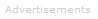# Definition of Equilibrium

What is Equilibrium?

A chemical reaction is in equilibrium when the concentrations of reactants and products are constant - their ratio does not vary.Equilibrium happens when a chemical reaction does not convert all reactants to products: many reactions reach a state of balance or dynamic equilibrium in which both reactants and products are present.

Another way of defining equilibrium is to say that a system is in equilibrium when the forward and reverse reactions occur at equal rates.

Equilibrium does not necessarily mean that reactants and products are present in equal amounts. It means that the reaction has reached a point where the concentrations of the reactant and product are unchanging with time, because the forward and backward reactions have the same rate.

Equilibrium Symbol

Chemists draw arrows that look like this:

to show a reaction or system is in equilibrium. In the simple chemical equation below reactant A is in equilibrium with product B.

A ⇌ B
A Simple Real Example

A common example of an equilibrium is when you saturate water with table salt - in other words, you add so much salt to the water that all of the salt cannot dissolve.

Although you may think nothing much is happening in this saturated solution, at the molecular level, there is constant activity, with sodium chloride dissolving and precipitating constantly.

If the temperature is constant, equilibrium will be reached so that for every sodium chloride that dissolves, another will precipitate. At this point the system is in equilibrium, and we can write:

NaCl(solid) ⇌ Na+(aq) + Cl-(aq)

The equilibrium constant indicates whether the forward reaction or backward reaction is more favored.

An equilibrium constant greater than one means the forward reaction is favored. An equilibrium constant equal to one means the forward reaction and backward reactions are equally favored. An equilibrium constant less than one means the backward reaction is favored.Search the Dictionary for More Terms